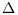Scilab Home page | Wiki | Bug tracker | Forge | Mailing list archives | ATOMS | File exchange
Change language to: English - Português - 日本語 - Русский

See the recommended documentation of this function

# mucomp

mu (structured singular value) calculation

### Syntax

`[BOUND, D, G] = mucomp(Z, K, T)`

### Arguments

Z

the complex n-by-n matrix for which the structured singular value is to be computed

K

the vector of length m containing the block dimensions of the structured uncertainty. The uncertaintyis supposed to be a block diagonal matrix.

T

the vector of length m indicating the type of each uncertainty block. T(I) = 1 if the corresponding block is real T(I) = 2 if the corresponding block is complex.

BOUND

the upper bound on the structured singular value.

D, G

vectors of length n containing the diagonal entries of the diagonal matrices D and G, respectively, such that the matrix ```Z'*diag(D)^2*Z + sqrt(-1)*(diag(G)*Z-Z'*diag(G)) - bound^2*diag(D)^2``` is negative semidefinite.

### Description

This function computes an upper bound on the structured singular value for a given square complex matrix and given block structure of the uncertainty.

The structured singular valueis defined as the inverse of the norm of the smallest uncertaintythat makes. Hereis supposed to be a block diagonal matrix.

### Examples

```K=[1,1,2,1,1];
T=[1,1,2,2,2];
Z=[-1+%i*6, 2-%i*3, 3+%i*8, 3+%i*8,-5-%i*9,-6+%i*2;
4+%i*2,-2+%i*5,-6-%i*7,-4+%i*11,8-%i*7, 12-%i;
5-%i*4,-4-%i*8, 1-%i*3,-6+%i*14,2-%i*5, 4+%i*16;
-1+%i*6, 2-%i*3, 3+%i*8, 3+%i*8,-5-%i*9,-6+%i*2;
4+%i*2,-2+%i*5,-6-%i*7,-4+%i*11,8-%i*7, 12-%i;
5-%i*4,-4-%i*8, 1-%i*3,-6+%i*14,2-%i*5, 4+%i*16];

[BOUND, D, G] = mucomp(Z, K, T)
spec(Z'*(diag(D)^2)*Z + %i*(diag(G)*Z-Z'*diag(G)) - BOUND^2*diag(D)^2)```

### Used functions

This function is based on the Slicot routine AB13MD.

### References

Fan, M.K.H., Tits, A.L., and Doyle, J.C. Robustness in the presence of mixed parametric uncertainty and unmodeled dynamics. IEEE Trans. Automatic Control, vol. AC-36, 1991, pp. 25-38. Slicot routine AB13MD.